Plot each trajectory within a viewr object

## Usage

``````plot_viewr_trajectories(
obj_name,
plot_axes = c("length", "width"),
multi_plot = FALSE
)``````

## Arguments

obj_name

A viewr object (a tibble or data.frame with attribute `pathviewr_steps` that includes `"viewr"`) that has been passed through `separate_trajectories()` or `get_full_trajectories()`.

plot_axes

Which position axes should be plotted? A character vector including exactly two of the following choices must be supplied: `length`, `width`, `height`. Default is c("length", "width").

multi_plot

Should separate plots (one per trajectory) be created or should one multi-plot grid be generated. Defaults to FALSE, which produces separate plots.

## Value

A (base-R) series of plots or single plot (if ```multi_plot = TRUE```) that depict each trajectory along the chosen axes.

Other plotting functions: `plot_by_subject()`, `visualize_frame_gap_choice()`

Vikram B. Baliga

## Examples

``````library(pathviewr)

## Import the example Motive data included in the package
motive_data <-
package = 'pathviewr'))

motive_full <-
motive_data %>%
clean_viewr(desired_percent = 50,
max_frame_gap = "autodetect",
span = 0.95)
#> autodetect is an experimental feature -- please report issues.

plot_viewr_trajectories(motive_full, multi_plot = FALSE)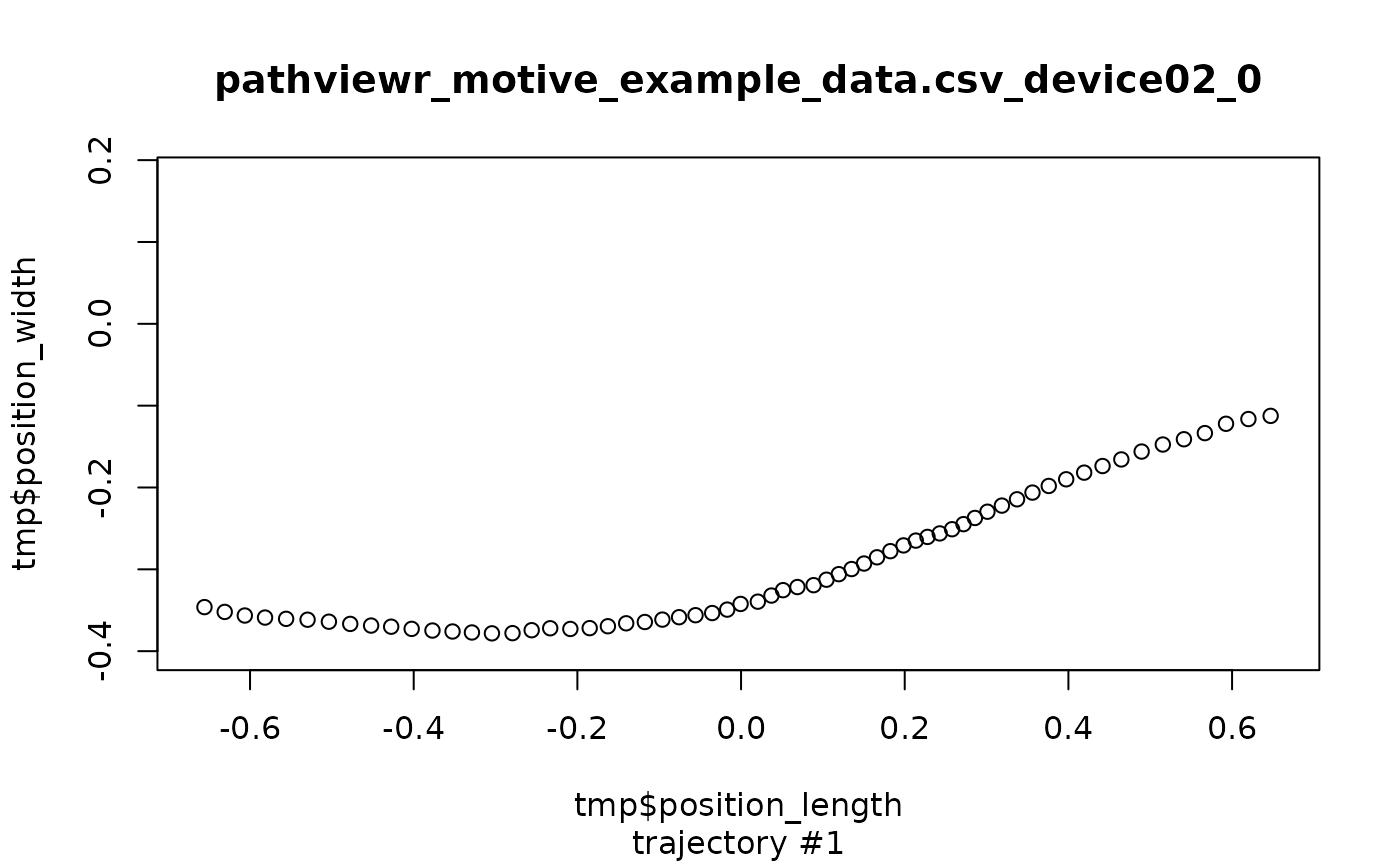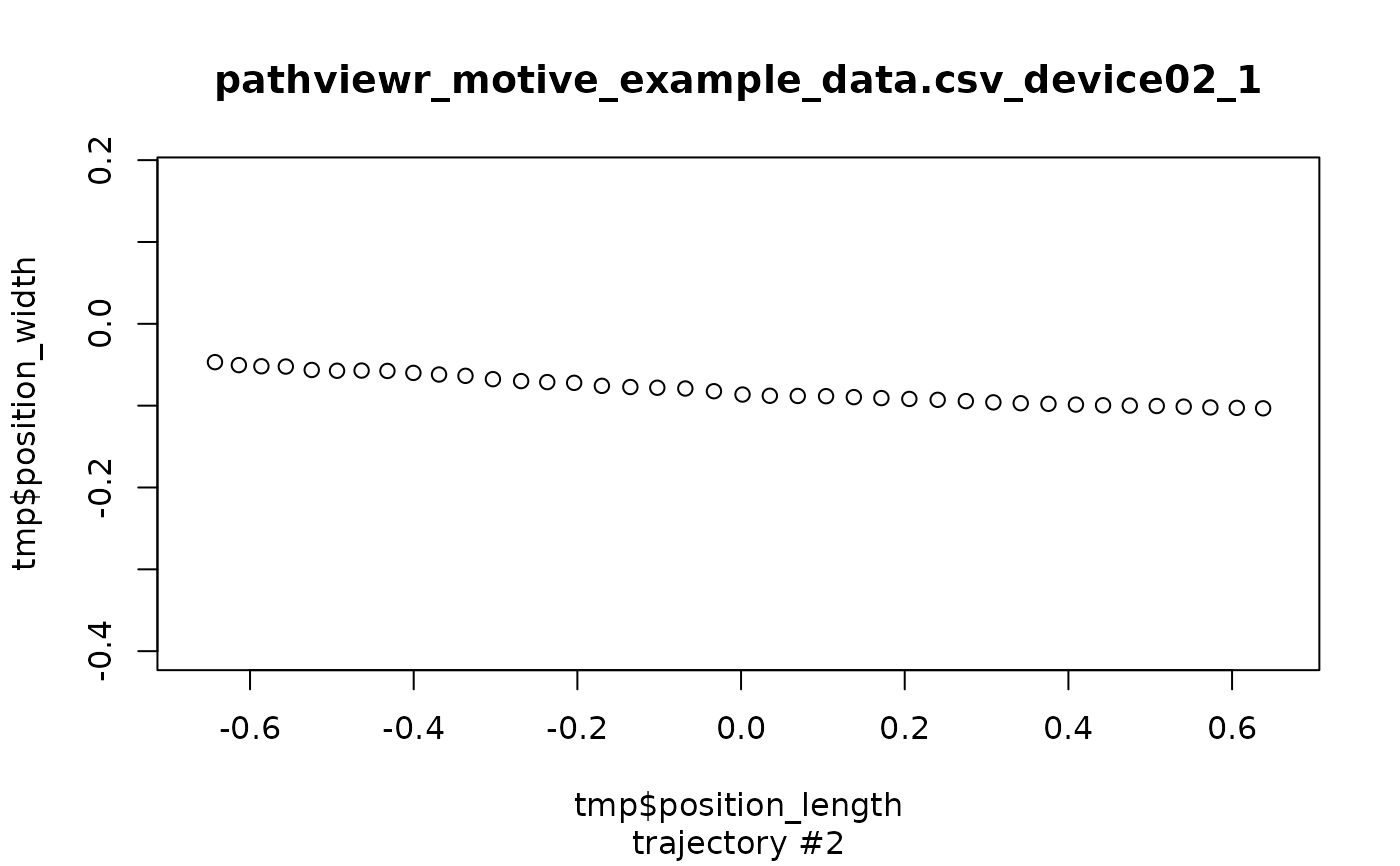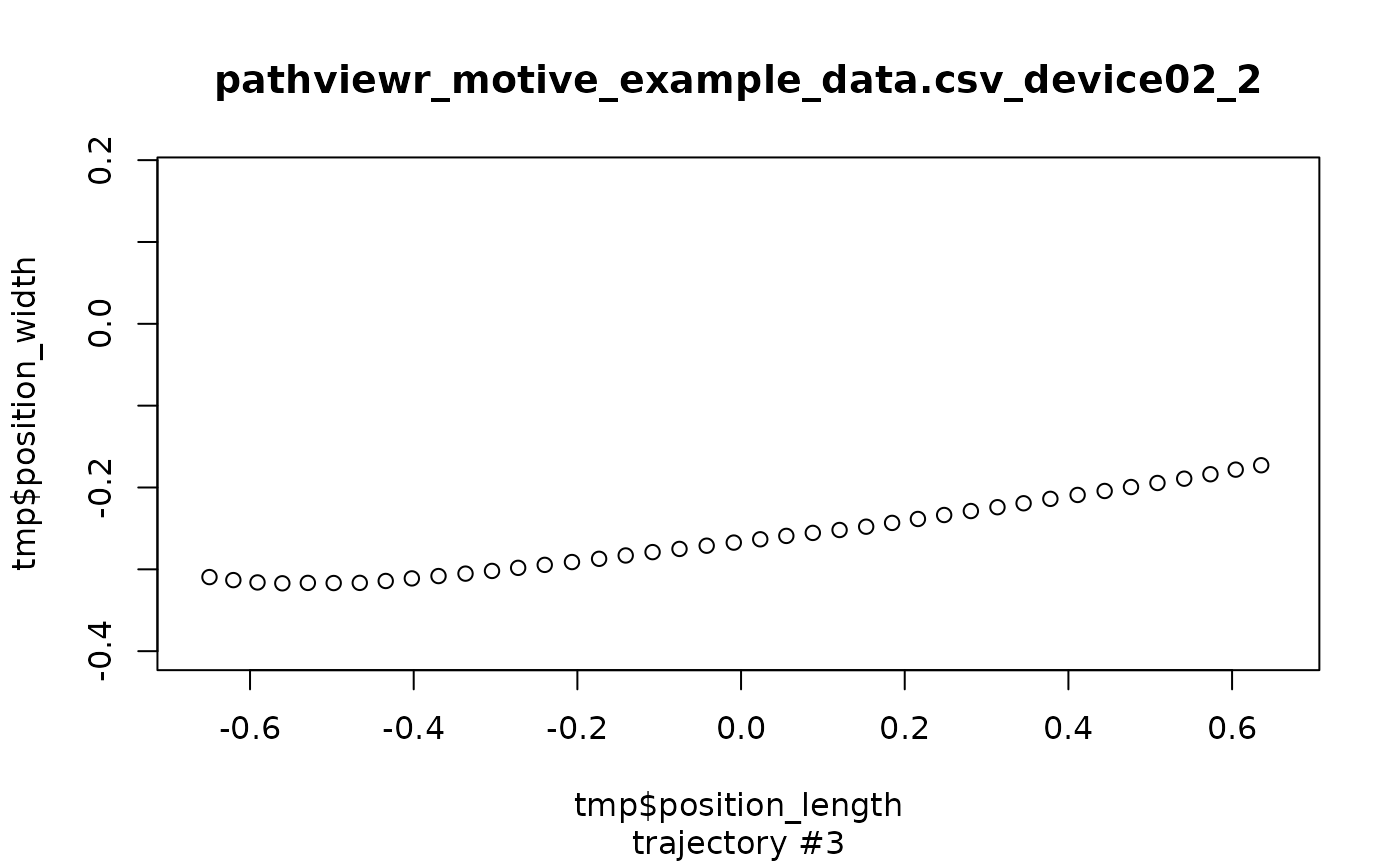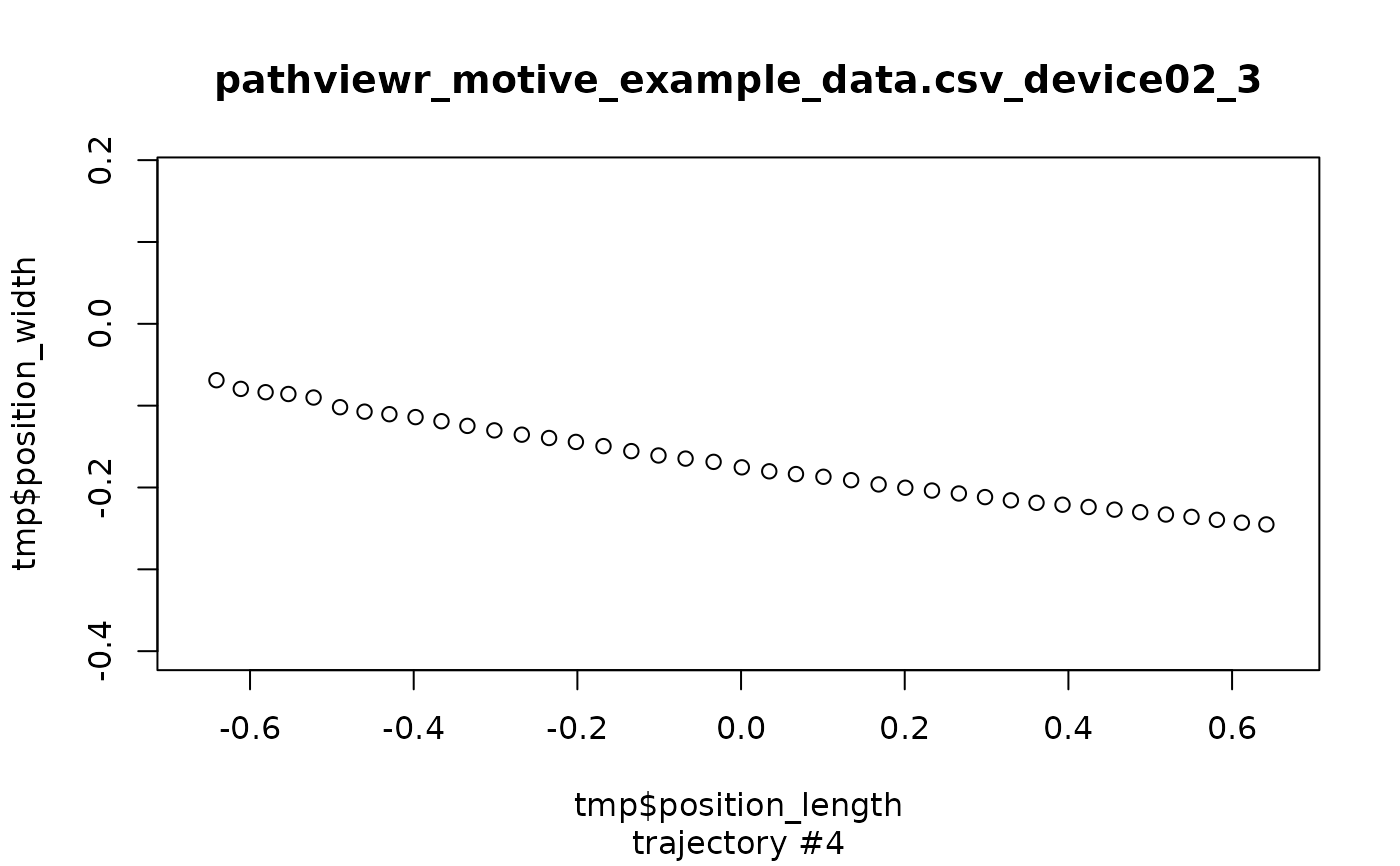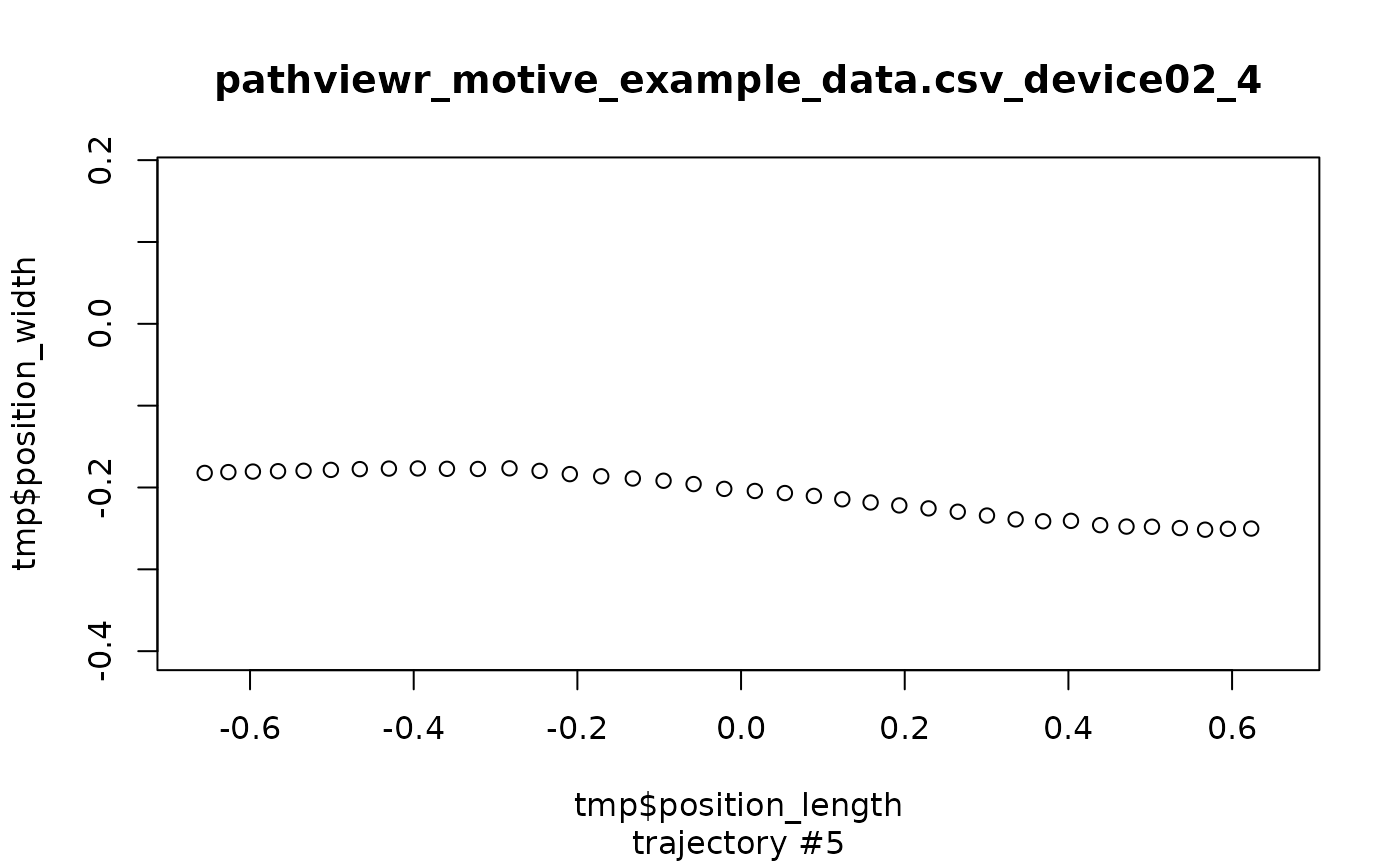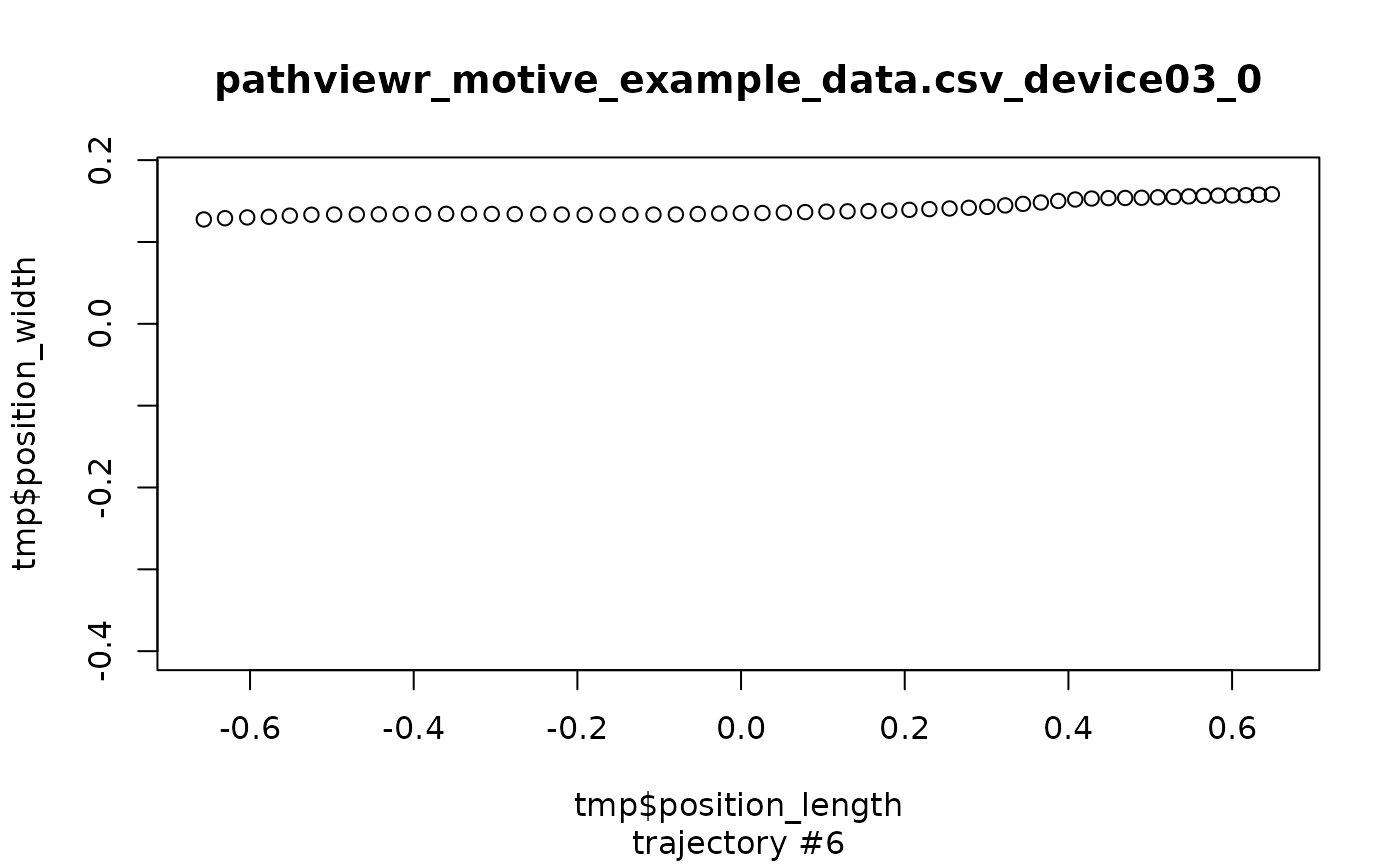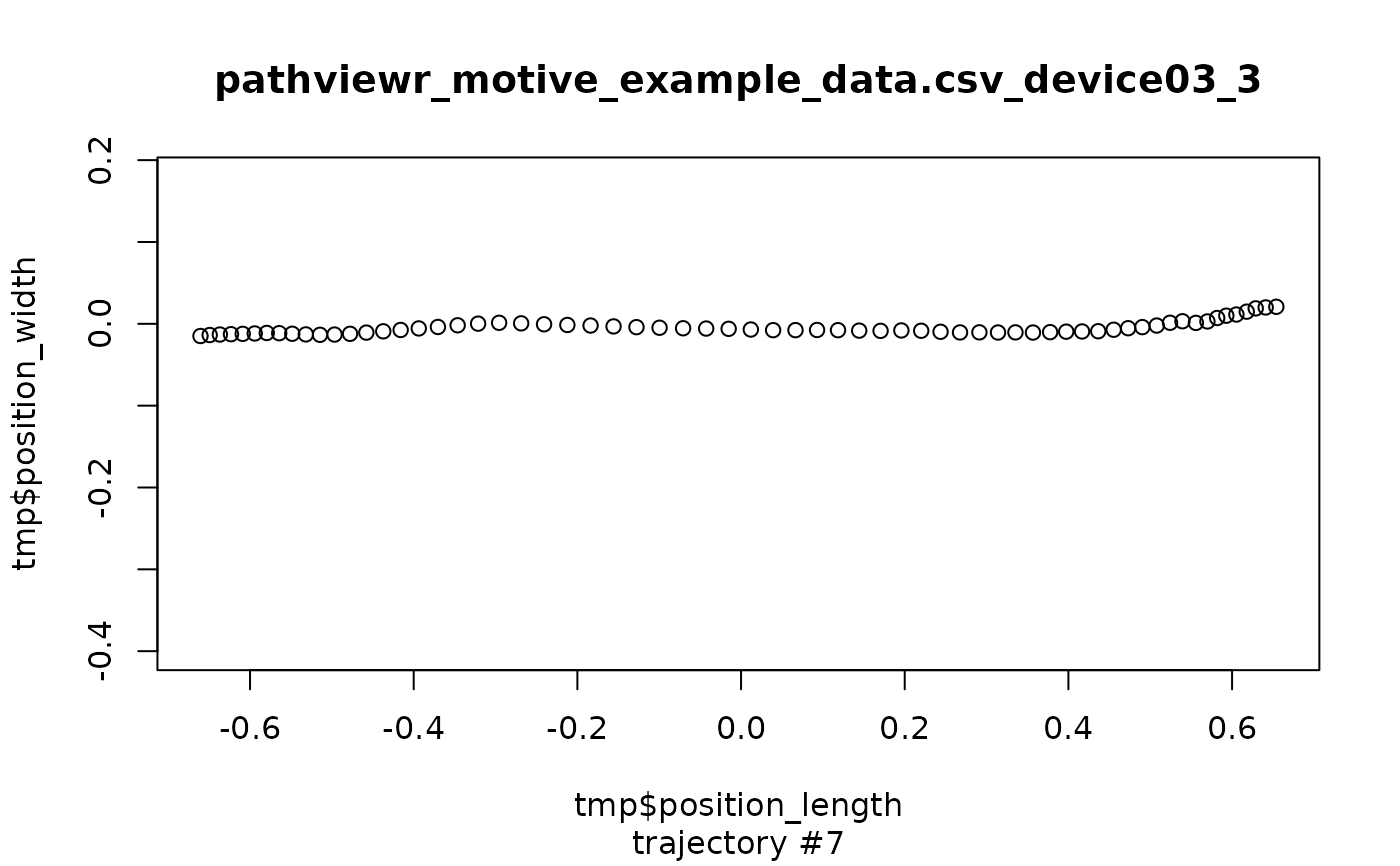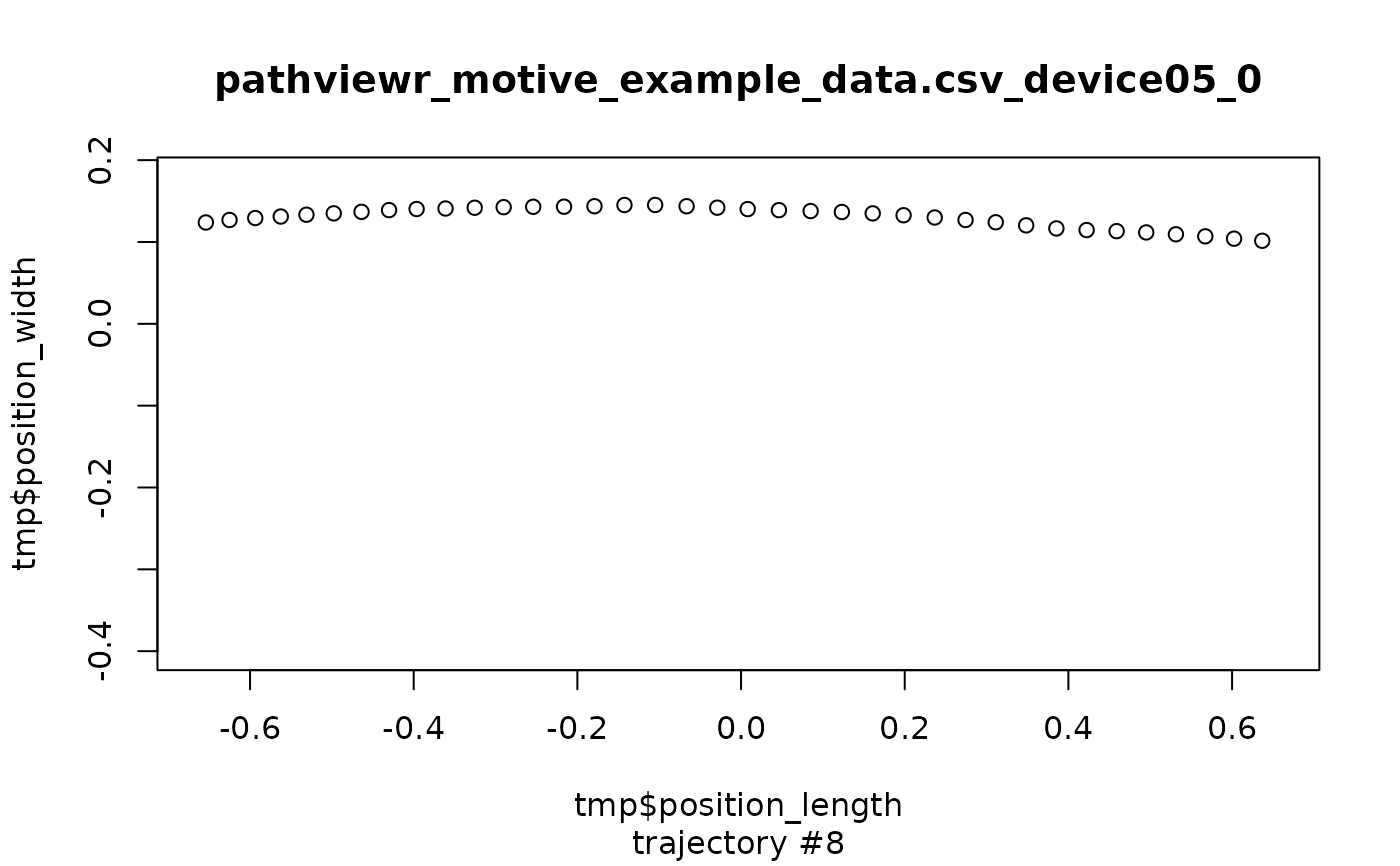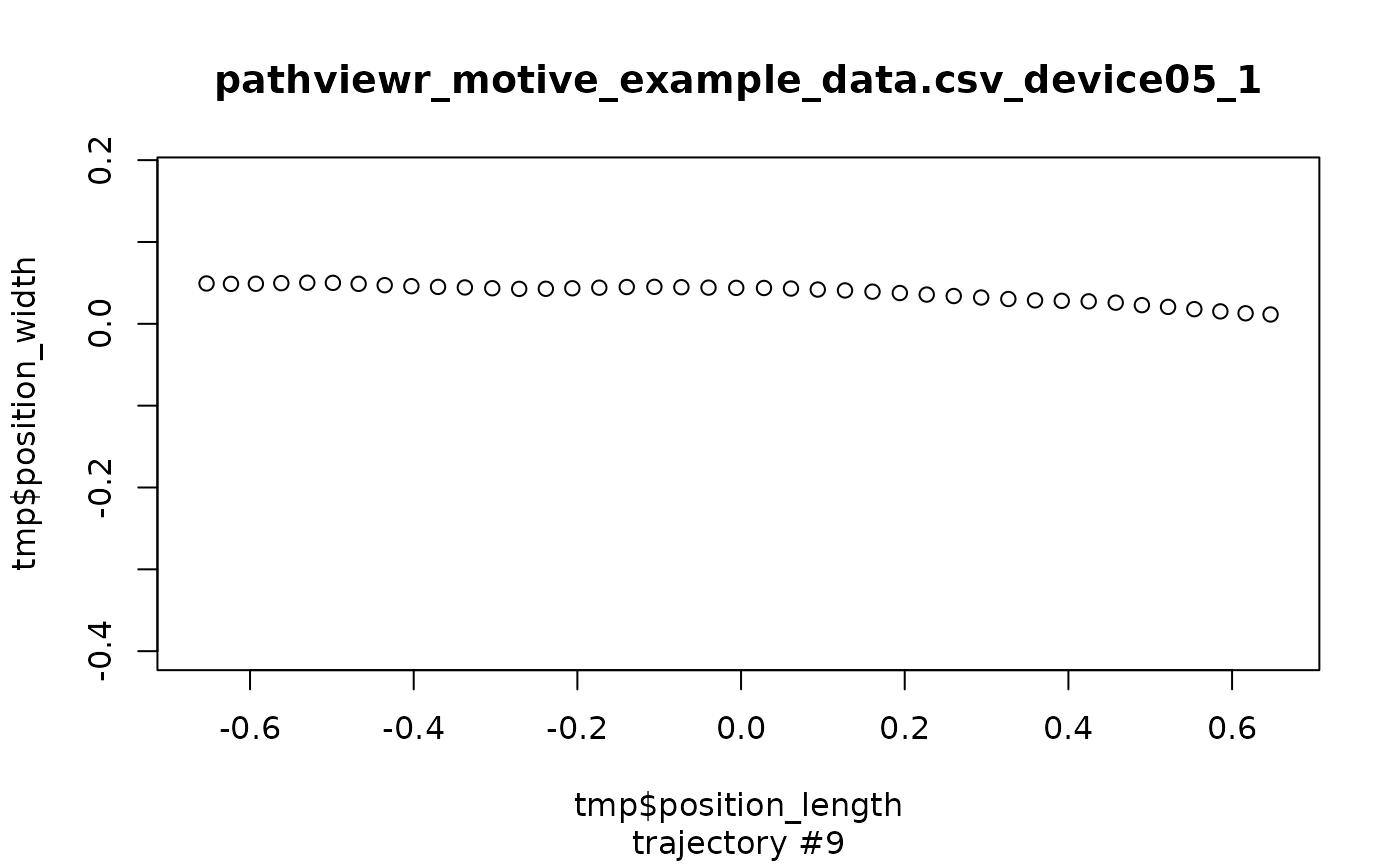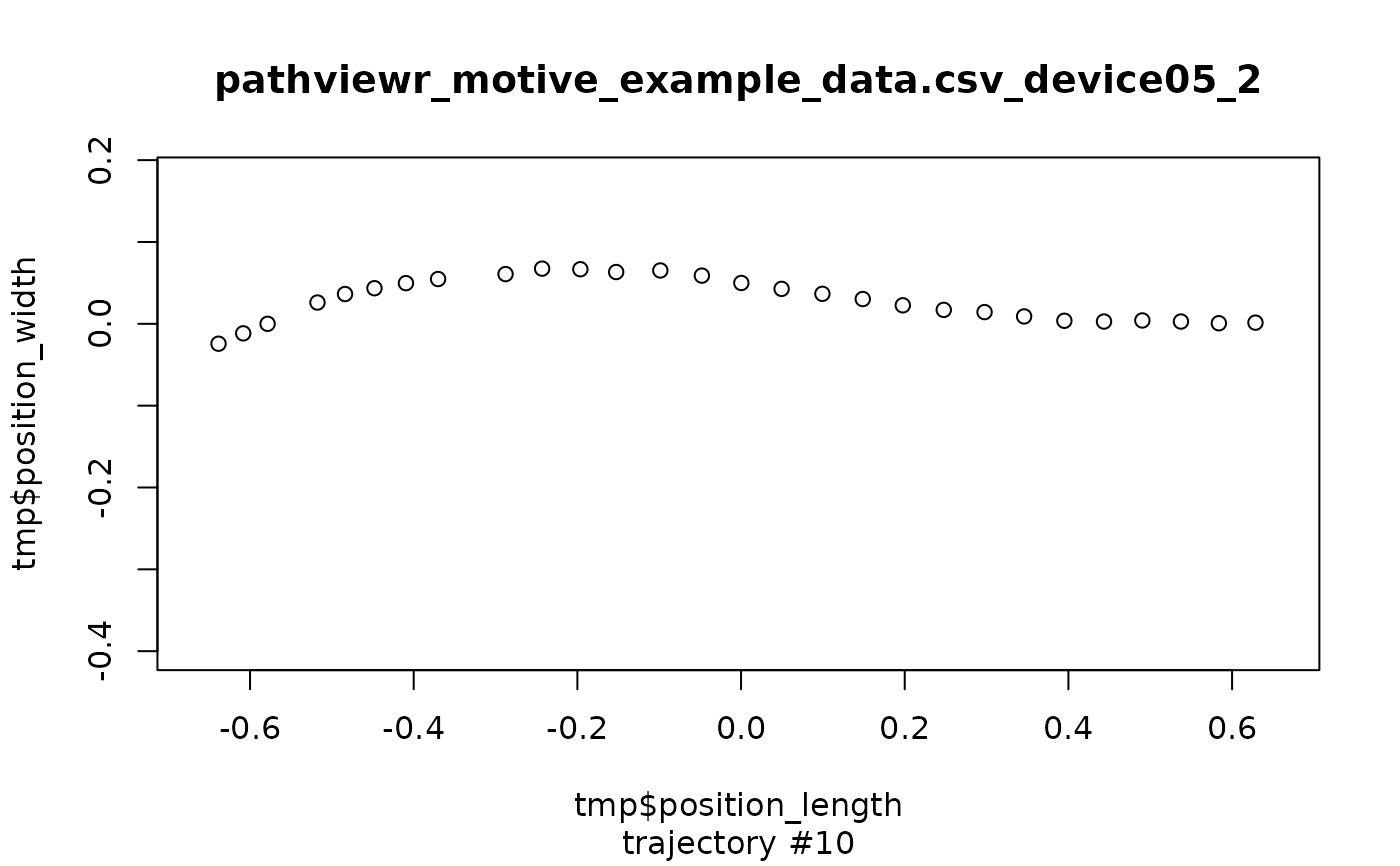plot_viewr_trajectories(motive_full, multi_plot = TRUE)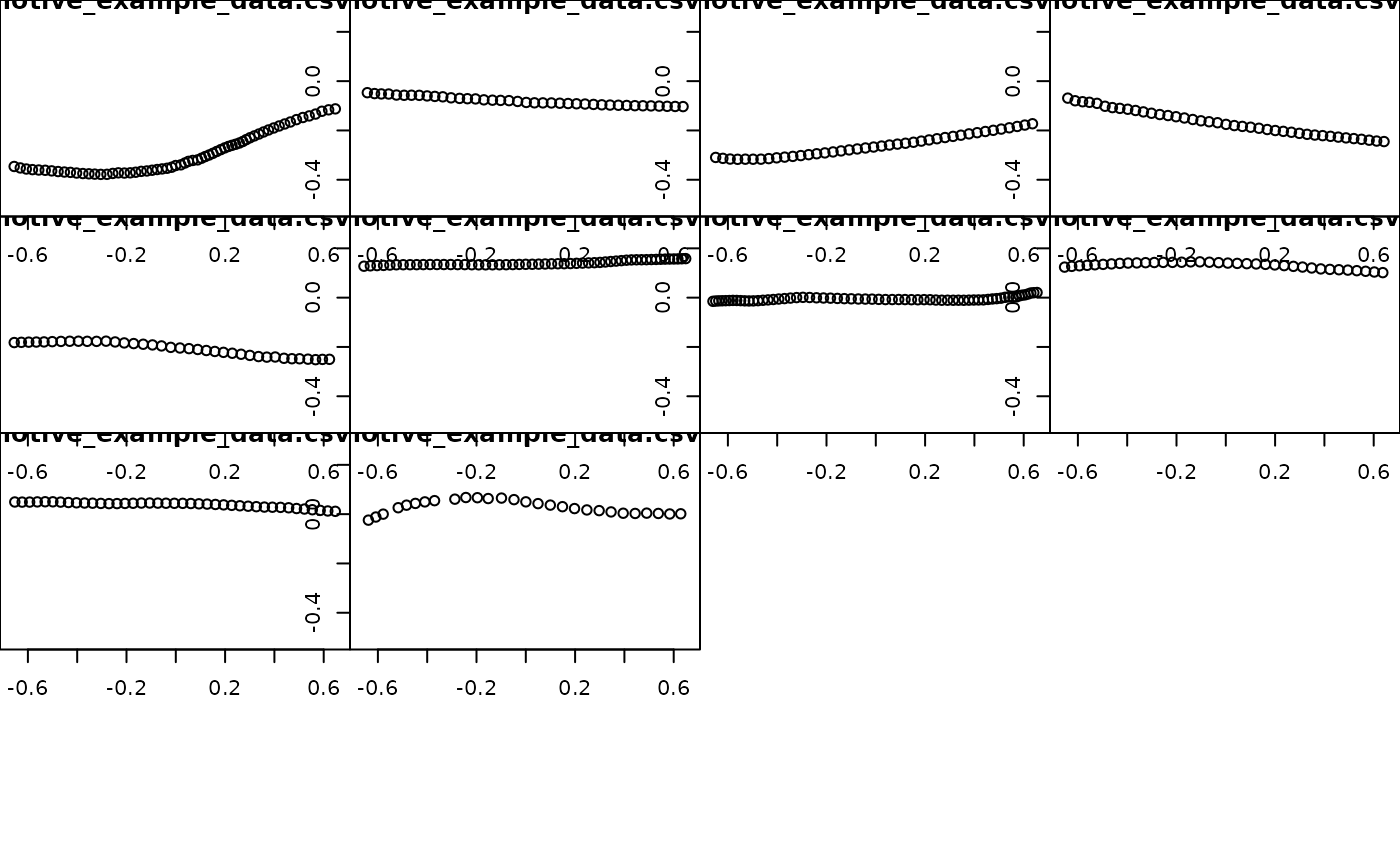``````